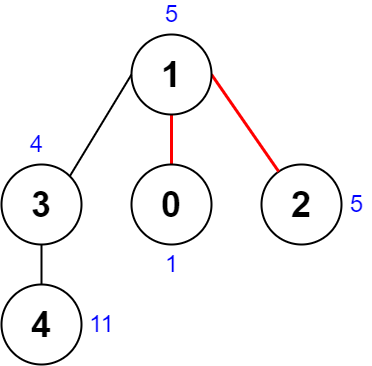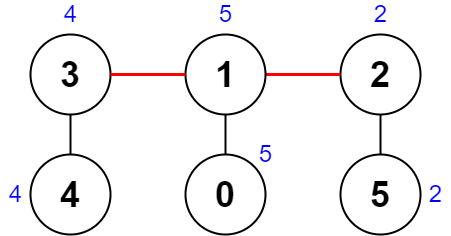##### Welcome to Subscribe On Youtube

Formatted question description: https://leetcode.ca/all/2322.html

# 2322. Minimum Score After Removals on a Tree

• Difficulty: Hard.
• Related Topics: Array, Bit Manipulation, Tree, Depth-First Search.
• Similar Questions: .

## Problem

There is an undirected connected tree with n nodes labeled from 0 to n - 1 and n - 1 edges.

You are given a 0-indexed integer array nums of length n where nums[i] represents the value of the ith node. You are also given a 2D integer array edges of length n - 1 where edges[i] = [ai, bi] indicates that there is an edge between nodes ai and bi in the tree.

Remove two distinct edges of the tree to form three connected components. For a pair of removed edges, the following steps are defined:

• Get the XOR of all the values of the nodes for each of the three components respectively.

• The difference between the largest XOR value and the smallest XOR value is the score of the pair.

• For example, say the three components have the node values: [4,5,7], [1,9], and [3,3,3]. The three XOR values are 4 ^ 5 ^ 7 = **6**, 1 ^ 9 = **8**, and 3 ^ 3 ^ 3 = **3**. The largest XOR value is 8 and the smallest XOR value is 3. The score is then 8 - 3 = 5.

Return the **minimum score of any possible pair of edge removals on the given tree**.

Example 1:Input: nums = [1,5,5,4,11], edges = [[0,1],[1,2],[1,3],[3,4]]
Output: 9
Explanation: The diagram above shows a way to make a pair of removals.
- The 1st component has nodes [1,3,4] with values [5,4,11]. Its XOR value is 5 ^ 4 ^ 11 = 10.
- The 2nd component has node  with value . Its XOR value is 1 = 1.
- The 3rd component has node  with value . Its XOR value is 5 = 5.
The score is the difference between the largest and smallest XOR value which is 10 - 1 = 9.
It can be shown that no other pair of removals will obtain a smaller score than 9.


Example 2:Input: nums = [5,5,2,4,4,2], edges = [[0,1],[1,2],[5,2],[4,3],[1,3]]
Output: 0
Explanation: The diagram above shows a way to make a pair of removals.
- The 1st component has nodes [3,4] with values [4,4]. Its XOR value is 4 ^ 4 = 0.
- The 2nd component has nodes [1,0] with values [5,5]. Its XOR value is 5 ^ 5 = 0.
- The 3rd component has nodes [2,5] with values [2,2]. Its XOR value is 2 ^ 2 = 0.
The score is the difference between the largest and smallest XOR value which is 0 - 0 = 0.
We cannot obtain a smaller score than 0.


Constraints:

• n == nums.length

• 3 <= n <= 1000

• 1 <= nums[i] <= 108

• edges.length == n - 1

• edges[i].length == 2

• 0 <= ai, bi < n

• ai != bi

• edges represents a valid tree.

## Solution

• class Solution {
private int ans = Integer.MAX_VALUE;

// function to travel 2nd time on the tree and find the second edge to be removed
private int helper(
int src, ArrayList<Integer>[] graph, int[] arr, int par, int block, int xor1, int tot) {
// Setting the value for the current subtree's XOR value
int myXOR = arr[src];
for (int nbr : graph[src]) {
// If the current nbr is niether the parent of this node nor the blocked node  , then
// only we'll proceed
if (nbr != par && nbr != block) {
int nbrXOR = helper(nbr, graph, arr, src, block, xor1, tot);
// 'src <----> nbr' is the second edge to be removed
// Getting the XOR value of the current neighbor
int xor2 = nbrXOR;
// The XOR of the remaining component
int xor3 = (tot ^ xor1) ^ xor2;
// Getting the minimum of the three values
int max = Math.max(xor1, Math.max(xor2, xor3));
// Getting the maximum of the three value
int min = Math.min(xor1, Math.min(xor2, xor3));
ans = Math.min(ans, max - min);
// Including the neighbour subtree's XOR value in the XOR value of the subtree
// rooted at src node
myXOR ^= nbrXOR;
}
}
// Returing the XOR value of the current subtree rooted at the src node
return myXOR;
}

// function to travel 1st time on the tree and find the first edge to be removed and
// then block the node at which the edge ends to avoid selecting the same node again
private int dfs(int src, ArrayList<Integer>[] graph, int[] arr, int par, int tot) {
// Setting the value for the current subtree's XOR value
int myXOR = arr[src];
for (int nbr : graph[src]) {
// If the current nbr is not the parent of this node, then only we'll proceed
if (nbr != par) {
// After selecting 'src <----> nbr' as the first edge, we block 'nbr' node and then
// make a call to try all the second edges
int nbrXOR = dfs(nbr, graph, arr, src, tot);
// Calling the helper to find the try all the second edges after blocking the
// current node
helper(0, graph, arr, -1, nbr, nbrXOR, tot);
// Including the neighbour subtree's XOR value in the XOR value of the subtree
// rooted at src node
myXOR ^= nbrXOR;
}
}
// Returing the XOR value of the current subtree rooted at the src node
return myXOR;
}

public int minimumScore(int[] arr, int[][] edges) {
int n = arr.length;
ArrayList<Integer>[] graph = new ArrayList[n];
int tot = 0;
for (int i = 0; i < n; i++) {
// Initializing the graph and finding the total XOR
graph[i] = new ArrayList<>();
tot ^= arr[i];
}
for (int[] edge : edges) {
int u = edge;
int v = edge;
}
ans = Integer.MAX_VALUE;
dfs(0, graph, arr, -1, tot);
return ans;
}
}

############

class Solution {
private int s;
private int s1;
private int n;
private int ans = Integer.MAX_VALUE;
private int[] nums;
private List<Integer>[] g;

public int minimumScore(int[] nums, int[][] edges) {
n = nums.length;
g = new List[n];
this.nums = nums;
Arrays.setAll(g, k -> new ArrayList<>());
for (int[] e : edges) {
int a = e, b = e;
}
for (int v : nums) {
s ^= v;
}
for (int i = 0; i < n; ++i) {
for (int j : g[i]) {
s1 = dfs(i, -1, j);
dfs2(i, -1, j);
}
}
return ans;
}

private int dfs(int i, int fa, int x) {
int res = nums[i];
for (int j : g[i]) {
if (j != fa && j != x) {
res ^= dfs(j, i, x);
}
}
return res;
}

private int dfs2(int i, int fa, int x) {
int res = nums[i];
for (int j : g[i]) {
if (j != fa && j != x) {
int a = dfs2(j, i, x);
res ^= a;
int b = s1 ^ a;
int c = s ^ s1;
int t = Math.max(Math.max(a, b), c) - Math.min(Math.min(a, b), c);
ans = Math.min(ans, t);
}
}
return res;
}
}

• class Solution:
def minimumScore(self, nums: List[int], edges: List[List[int]]) -> int:
def dfs(i, fa, x):
res = nums[i]
for j in g[i]:
if j != fa and j != x:
res ^= dfs(j, i, x)
return res

def dfs2(i, fa, x):
nonlocal s, s1, ans
res = nums[i]
for j in g[i]:
if j != fa and j != x:
a = dfs2(j, i, x)
res ^= a
b = s1 ^ a
c = s ^ s1
t = max(a, b, c) - min(a, b, c)
ans = min(ans, t)
return res

g = defaultdict(list)
for a, b in edges:
g[a].append(b)
g[b].append(a)

s = 0
for v in nums:
s ^= v
n = len(nums)
ans = inf
for i in range(n):
for j in g[i]:
s1 = dfs(i, -1, j)
dfs2(i, -1, j)
return ans

############

# 2322. Minimum Score After Removals on a Tree
# https://leetcode.com/problems/minimum-score-after-removals-on-a-tree

class Solution:
def minimumScore(self, nums: List[int], edges: List[List[int]]) -> int:
N, M = len(nums), len(edges)

graph = defaultdict(list)
children = defaultdict(set)
degree =  * N
xor = nums.copy()

for a, b in edges:
graph[a].append(b)
graph[b].append(a)
degree[a] += 1
degree[b] += 1

V = 0
dq = deque()
seen = set()

for node in range(N):
V ^= nums[node]

if degree[node] == 1:
dq.append(node)

while dq:
node = dq.popleft()

for nei in graph[node]:
if nei not in seen:
children[nei] |= children[node]
xor[nei] ^= xor[node]

degree[nei] -= 1

if degree[nei] == 1 and len(seen) != N - 1:
dq.append(nei)

res = float('inf')

for i in range(M - 1):
for j in range(i + 1, M):
a, b = edges[i]
if b in children[a]: a, b = b, a

c, d = edges[j]
if d in children[c]: c, d = d, c

if a in children[c]:
s = [xor[a], xor[c] ^ xor[a], V ^ xor[c]]
elif c in children[a]:
s = [xor[c], xor[a] ^ xor[c], V ^ xor[a]]
else:
s = [xor[c], xor[a], V ^ xor[c] ^ xor[a]]

res = min(res, max(s) - min(s))

return res


• class Solution {
public:
vector<int> nums;
int s;
int s1;
int n;
int ans = INT_MAX;
vector<vector<int>> g;

int minimumScore(vector<int>& nums, vector<vector<int>>& edges) {
n = nums.size();
g.resize(n, vector<int>());
for (auto& e : edges) {
int a = e, b = e;
g[a].push_back(b);
g[b].push_back(a);
}
for (int& v : nums) s ^= v;
this->nums = nums;
for (int i = 0; i < n; ++i) {
for (int j : g[i]) {
s1 = dfs(i, -1, j);
dfs2(i, -1, j);
}
}
return ans;
}

int dfs(int i, int fa, int x) {
int res = nums[i];
for (int j : g[i])
if (j != fa && j != x) res ^= dfs(j, i, x);
return res;
}

int dfs2(int i, int fa, int x) {
int res = nums[i];
for (int j : g[i])
if (j != fa && j != x) {
int a = dfs2(j, i, x);
res ^= a;
int b = s1 ^ a;
int c = s ^ s1;
int t = max(max(a, b), c) - min(min(a, b), c);
ans = min(ans, t);
}
return res;
}
};

• func minimumScore(nums []int, edges [][]int) int {
n := len(nums)
g := make([][]int, n)
for _, e := range edges {
a, b := e, e
g[a] = append(g[a], b)
g[b] = append(g[b], a)
}
s := 0
for _, v := range nums {
s ^= v
}
s1 := 0
ans := math.MaxInt32
var dfs func(int, int, int) int
var dfs2 func(int, int, int) int
dfs = func(i, fa, x int) int {
res := nums[i]
for _, j := range g[i] {
if j != fa && j != x {
res ^= dfs(j, i, x)
}
}
return res
}
dfs2 = func(i, fa, x int) int {
res := nums[i]
for _, j := range g[i] {
if j != fa && j != x {
a := dfs2(j, i, x)
res ^= a
b := s1 ^ a
c := s ^ s1
t := max(max(a, b), c) - min(min(a, b), c)
ans = min(ans, t)
}
}
return res
}
for i := 0; i < n; i++ {
for _, j := range g[i] {
s1 = dfs(i, -1, j)
dfs2(i, -1, j)
}
}
return ans
}

func max(a, b int) int {
if a > b {
return a
}
return b
}

func min(a, b int) int {
if a < b {
return a
}
return b
}


Explain:

nope.

Complexity:

• Time complexity : O(n).
• Space complexity : O(n).